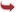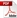International Journal of Scientific and Research Publications

#### IJSRP, Volume 6, Issue 6, June 2016 Edition [ISSN 2250-3153]Optimal Linear Control of an Energy Harvesting System
Ukoima Kelvin Nkalo
Abstract: This paper presents an optimal linear control of an energy harvesting system. Energy harvesting system models are typically non linear and therefore utilize a non linear controller. In this work, an investigation on the performance of a linear controller for a nonlinear harvester model is shown. The aim is to maximize the energy produced by implementing an optimal linear control algorithm. A mathematical model of the harvester is given. All simulations were conducted in matlab and simulink. Results obtained satisfy the conditions of optimality.
[VIEW FULL PAPER][DOWNLOAD]

Reference this Research Paper (copy & paste below code):

Ukoima Kelvin Nkalo (2018); Optimal Linear Control of an Energy Harvesting System; Int J Sci Res Publ 6(6) (ISSN: 2250-3153). http://www.ijsrp.org/research-paper-0616.php?rp=P545498